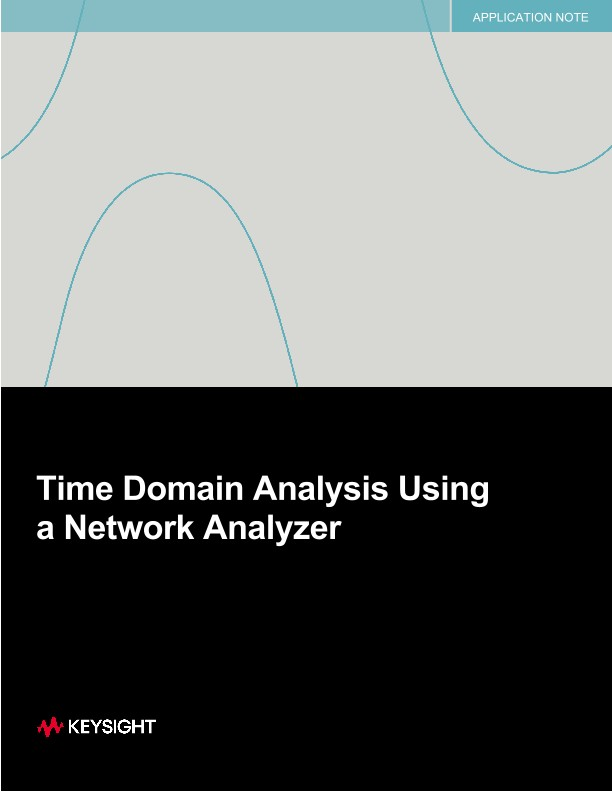# Time Domain Analysis Using a Network Analyzer

アプリケーションノート

Introduction

Time-domain analysis is useful for measuring impedance values along a transmission line and for evaluating a device problem (discontinuity) in time or distance. Time-domain display provides a more intuitive and direct look at the device under test (DUT) characteristics. In addition, it gives more meaningful information concerning the broadband response of a transmission system than other measuring techniques by showing the effect of each discontinuity as a function of time or distance. This document will focus on time-domain analysis (displays) generated from vector network analyzers (VNAs). The intent is to provide engineers with the frequency domain background, an in-depth view of how a time-domain display is created from frequency domain data (S-parameters), and how to apply the time domain display to common problems in RF systems. Keysight Technologies offers other documents that cover in detail the use of time-domain displays (using data captured with either a time-domain reflectometer (oscilloscope) or VNA). See the bibliography for more details.

• Introduction
• Time-domain and the Network Analyzer
• Effects of discrete sampling
• Time-domain modes with VNA
• Windowing
• Gating
• Measurement Range
• Resolution
• Conclusion
• Appendix A
• Appendix B
• Bibliography

A note on naming conventions

The term time domain can mean different things, depending on the context. For the purposes of this application note, the following conventions will be used:

Time-domain: refers to time domain analysis or display(s), as defined by an X-Y graph where the X-axis is either distance (electrical length) or time and the Y-axis is magnitude (typically impedance or voltage).

Time-domain reflectometry (TDR): refers to the method of measurement using a fast step generator and a receiver to measure either transmission or reflection. TDR is the common name for an oscilloscope with this capability. Note that a TDR with appropriate software can generate S-parameters (see Figure 1).

Vector network analyzer (VNA): refers to ratioed measurements where a swept CW source is tracked by a reflection or transmission receiver and results are typically displayed as S-parameters. This document will focus on how S-parameter data captured using a VNA can be transformed into a time-domain display.

History of TDR and time domain analysis

The measurement technique of time-domain reflectometry (TDR) was introduced in the early 1960s and works on the same principle as radar. A pulse of energy is transmitted down a cable (or other device - not necessarily a good conductor). When that pulse reaches the end of the cable or a fault along the cable, part or all of the pulse energy is reflected back to the instrument. TDR measurements are made by launching an impulse or a step into the test device and observing the response in time. Using a step generator and a broadband oscilloscope, a fast edge is launched into the transmission line. The incident and reflected voltage waves are monitored by the broadband oscilloscope at a particular point on the line. By measuring the ratio of the input voltage to the reflected voltage, the impedance of simple discontinuities can be calculated. The position of the discontinuity can also be calculated as a function of time by applying the velocity of propagation along the transmission line. The type of discontinuity (capacitive or inductive) can be identified by its response.

While the traditional TDR oscilloscope was useful as a qualitative tool, there were limitations that affected its accuracy and usefulness; a) TDR output step rise time – the spatial resolution of the measurement depends upon the step rise time; b) poor signal-to-noise ratio due to the wideband receiver architecture.

Then, in the 70’s, it was shown that the relationship between the frequency domain and the time domain could be described using the Fourier Transform. The Fourier Transform of the network reflection coefficient as a function of frequency is the reflection coefficient as a function of time; i.e., the distance along a transmission line. It was possible to measure the response of a DUT in the frequency domain and then mathematically calculate the inverse Fourier Transform of the data to give the time domain response.

A high-performance VNA combined with fast computation power created a unique measurement

capabilities. Using error-corrected data measured in the frequency domain, the response of a network to step and impulse time stimuli can be calculated and displayed as a function of time. This gives traditional time domain reflectometry capability in reflection and transmission and adds measurement capability of band-limited networks. By locating network elements in time and removing their effects from measured data, the vector network analyzer makes more precise frequency domain measurements possible.

Figure 1 shows how both time domain and frequency domain (S-parameter) displays can be generated by either a time-domain reflectometer (TDR) oscilloscope or a vector network analyzer (VNA). Data captured using either a TDR or VNA can be transformed into both displays.

×

ご希望の連絡方法をお知らせください。 *必須項目です。
ご登録のEメールアドレスの変更

ありがとうございました。

A sales representative will contact you soon.